## 线性规划问题

1. 确定决策变量。
2. 确定目标函数。
3. 找出约束条件。
4. 求最优解。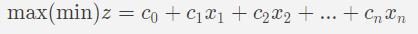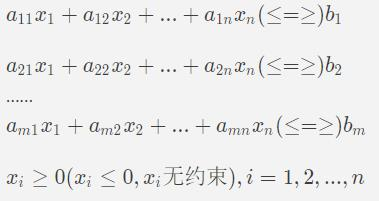• 变量满足约束条件的一组值成为线性规划问题的一个可行解。
• 所有可行解构成的集合成为线性规划的可行区域。
• 使目标函数取得极值的可行解称为最优解。
• 在最优解处目标函数的值成最优值

– 有唯一最优解。
– 有无数多个最优解。
– 没有最优解。

### 线性规划的标准型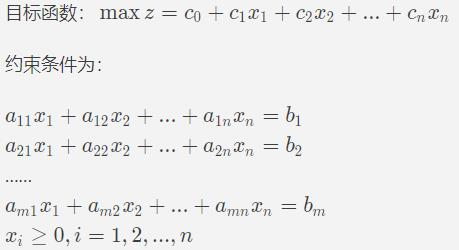#### 四个要求：

1. 目标函数为最大值（即为max）；
2. 约束条件常数项bi>=0；
3. 约束条件全部为等式约束；
4. 决策变量xi非负约束。

1. 一般线性规划行驶中目标函数如果求最小值，那么令z’=-z，得到最优解后，加负号即可。
2. 右端常数项小于零时，则不等式两边同乘-1，将其变成大于零；同时改变不等号的方向，保证恒等变形。
3. 约束条件为大于与等于约束条件时，则在不等式左侧减去一个新的非负变量将不等式约束改为等式约束。例如2x1-3x2≥10，修改为：2x1-3x2-x3=10，x3>0。
4. 约束条件为小于等于约束时，则在不等式左侧加去一个新的非负变量将不等式约束改为等式约束。
5. 无约束的决策变量x，即可正可负的变量，则引入两个新的非负变量x’和x”，令x-x’-x”，其中x’≥0，x”≥0，将x带入线性规划模型。例如：2x1-3x2+x3=10，x3无约束，则可以修改为：x3=x4-x5，x4>0,x5>0，带入方程得到2x1-3x2+x4-x5=10，x4>0,x5>0
注意：引入的新的非负变量称为松弛变量

### 单纯形算法图解

1. 建立初始单纯形表。找出基本变量和非基本变量，将目标函数由非基本变量表示，建立初始单纯形表。注意：如果目标函数含有基本变量，要通过约束条件方程转换为非基本变量。 还要注意：基本变量的系数要转化为1 ，否则不能按照下面的计算方法。基本变量做行，非基本变量做列，检验数放第一行，常数项放低1列，约束条件中非基本变量的系数作为值，构造初始单纯形表。如下所示：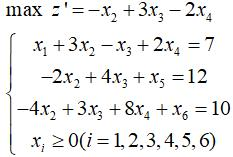bx2x3x4
c0-13-2
x173-12
x512-240
x610-438
1. 判断是否得到最优解。判别并检查目标函数的所有系数。
• 如果所有的cj<=0，则已经获得最优解，算法结束。
• 若在检验数cj中，有些为正数，但其中某一正的检验数所对应的列向量的各分量均小于等于0，则线性规划问题无界，算法结束。
• 若在检验数cj中，有些为正数且它们对应的列向量中有正的分量，则转到第3步。
2. 选入基变量。选取所有正检验数中最大的一个，记为ce，其对应的非基本变量为xe，称为入基变量，xe对应的列向量[a1e,a2e,…,ame]T为入基列。在本例中，x3为入基变量，x3对应的列向量为入基列，如下所示。

bx2x3x4
c0-13-2
x173-12
x512-240
x610-438
1. 选离基变量、选取“常数列元素/入基列元素”正比值最小者，所对应的基本变量xk为离基变量。xk对应的行向量为离基行。在本例中，x5为离基变量，对应的行向量为离基行。
2. 换基变换。在单纯形表上将入基变量和离基变量互换位置，即在本例中将x3和x5交换位置，换基变换后如下所示。
bx2x5x4
c0-13-2
x173-12
x312-240
x610-438
1. 计算新的单纯形表。按照下面的方法计算新的单纯形表，然后转第2步。4个特殊位置如下：
• 入基列：-原值/交叉位值（不包括交叉位）。
• 离基行=原值/交叉位值（不包括交叉位）
• 交叉位=原值取倒数。
• c0：原值+同行入基列元素*同列离基行元素/交叉位值。
• 一般位置元素=原值-同行入基列元素*同列离基行元素/交叉位值。
bx2x5x4
c90.5-0.75-2
x1102.50.252
x33-0.50.250
x61-2.5-0.758
1. 判断是否得到最优解，若没有继续第3~6步，直到找到最优解或判定无界解停止。在本例中，再次选定基列变量x2和离基变量x1，将他们互换位置，重新计算单纯形表，得到下面的表。
bx1x5x4
c11-0.2-0.8-2.4
x240.40.10.8
x350.20.30.4
x6111-0.510

## 工厂最大效益——单纯形算法

### 问题分析

x1：产品A的售出量。
x2：产品A在第二车间加工后售出量.
x3：产品B售出量。
x4：产品B在第三车间加工后售出量。
x5：第一车间所用原材料数量。

• 第一车间所有原材料费和人工费为：5x5+0.05×15x5=5.75x5（下面计算盈利时，均已除去第一车间的材料和人工费）

• A直接售出，盈利：10x1

• A加工后售出，因为有额外加工费、人工费：5+0.1×15=6.5，售价-额外成本=19-6.5=12.5，盈利12.5x2
• B直接售出，盈利16x3
• B加工后售出，额外费用4+0.08×15=5.2，售价-成本=24-5.2=18.8，盈利18.8x4
• 总盈利：z=10x1+12.5x2+16x3+18.8x4-5.75x5
• 所以目标函数和约束条件如下：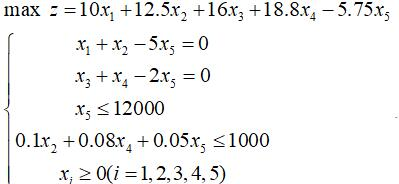### 完美图解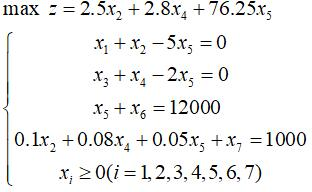bx2x4x5
c02.52.876.25
x1010-5
x3001-2
x612000001
x710000.10.080.05

### 代码实现

float kernel;//存储非单纯形表char FJL = {};//非基本变量char JL = {};//基本变量int n, m, i, j;

void print()//输出单纯型表{ cout<<endl; cout<<"----------单纯形表如下：----------"<<endl; cout<<" "; cout<<setw(7)<<"b "; for(i=1;i<=m;i++) cout<<setw(7)<<"x"<<FJL[i]; cout<<endl; cout<<"c "; for(i=0;i<=n;i++) { if(i>=1) cout<<"x"<<JL[i]; for(j=0;j<=m;j++) cout<<setw(7)<<kernel[i][j]<<" "; cout<<endl; }}void DCXA(){ float max1; //max1用于存放最大的检验数 float max2; //max2用于存放最大正检验数对应的基本变量的最大系数 int e=-1; //记录入基列 int k=-1; //记录离基行 float min; //循环迭代，直到找到问题的解或无解为止 while(true) { max1=0; min=100000000; for(j=1;j<=m;j++) //找入基列(最大正检验数对应的列) { if(max1<kernel[j]) { max1=kernel[j]; e=j; } } if(max1<=0) //最大值小于等于0，即所有检验数小于等于0，满足获得最优解的条件 { cout<<endl; cout<<"获得最优解:"<<kernel<< endl; print(); break; } for(j=1;j<=m;j++) //判断正检验数对应的列是否都小于等于0，如果是则无界解 { max2=0; if(kernel[j]>0) { for(i=1;i<=n;i++) //搜索正检验数对应的列 if(max2<kernel[i][j]) max2=kernel[i][j]; if(max2==0) { cout<<"解无界"<<endl; return;//退出函数，不能用break，因为它只是退出当前循环 } } } for(i=1;i<=n;i++) //找离基行(常数列/入基列正比值最小对应的行) { float temp=kernel[i]/kernel[i][e]; //常数项在前,temp=fabs(temp); if(temp>0&&temp<min) //找离基变量 { min=temp; k=i; } } cout<<"入基变量："<<"x"<<FJL[e]<<" "; cout<<"离基变量："<<"x"<<JL[k]<<endl; //换基变换(转轴变换) char temp=FJL[e]; FJL[e]=JL[k]; JL[k]=temp; for(i=0;i<=n;i++) //计算除入基列和出基行的所有位置的元素 { if(i!=k) { for(j=0;j<=m;j++) { if(j!=e) { if(i==0&&j==0) //计算特殊位c0,即目标函数的值 kernel[i][j]=kernel[i][j]+kernel[i][e]*kernel[k][j]/kernel[k][e]; else //一般位置 kernel[i][j]=kernel[i][j]-kernel[i][e]*kernel[k][j]/kernel[k][e]; } } } } for(i=0;i<=n;i++) //计算特殊位,入基列的元素 { if(i!=k) kernel[i][e]=-kernel[i][e]/kernel[k][e]; } for(j=0;j<=m;j++) //计算特殊位,离基行的元素 { if(j!=e) kernel[k][j]=kernel[k][j]/kernel[k][e]; } //计算特殊位,交叉位置 kernel[k][e]=1/kernel[k][e]; print(); }}

### 复杂度分析

1. 时间复杂度：在输入基本变量和非基本变量中用了n+m循环次数，在输入单纯形表时有nm此循环，打印最优解时有n+nm次的时间打印结果，在寻找入基列和离基行中，最坏情况下有O(n*m)次循环，在循环迭代中最坏情况需要2n迭代，则时间复杂度为O(2n)。
2. 空间复杂度：O(1)。